Hydrol. Earth Syst. Sci., 26, 1745–1754, 2022
https://doi.org/10.5194/hess-26-1745-2022
Hydrol. Earth Syst. Sci., 26, 1745–1754, 2022
https://doi.org/10.5194/hess-26-1745-2022
Research article | 06 Apr 2022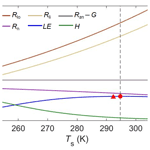# Testing a maximum evaporation theory over saturated land: implications for potential evaporation estimation

Testing a maximum evaporation theory over saturated land: implications for potential evaporation estimation
Zhuoyi Tu1, Yuting Yang1, and Michael L. Roderick2 Zhuoyi Tu et al.
• 1State Key Laboratory of Hydroscience and Engineering, Department of Hydraulic Engineering, Tsinghua University, Beijing, China
• 2Research School of Earth Sciences, Australian National University, Canberra, ACT, Australia

Correspondence: Yuting Yang (yuting_yang@tsinghua.edu.cn)

Abstract

State-of-the-art evaporation models usually assume net radiation (Rn) and surface temperature (Ts; or near-surface air temperature) to be independent forcings on evaporation. However, Rn depends directly on Ts via outgoing longwave radiation, and this creates a physical coupling between Rn and Ts that extends to evaporation. In this study, we test a maximum evaporation theory originally developed for the global ocean over saturated land surfaces, which explicitly acknowledges the interactions between radiation, Ts, and evaporation. Similar to the ocean surface, we find that a maximum evaporation (LEmax) emerges over saturated land that represents a generic trade-off between a lower Rn and a higher evaporation fraction as Ts increases. Compared with flux site observations at the daily scale, we show that LEmax corresponds well to observed evaporation under non-water-limited conditions and that the Ts value at which LEmax occurs also corresponds with the observed Ts. Our results suggest that saturated land surfaces behave essentially the same as ocean surfaces at timescales longer than a day and further imply that the maximum evaporation concept is a natural attribute of saturated land surfaces, which can be the basis of a new approach to estimating evaporation.

Share
1 Introduction

Potential evaporation (EP), defined as the rate of evaporation (E) that would occur under non-water-stressed conditions, determines the upper boundary of E over a specific land surface for a given meteorological forcing. Although EP is more of a hypothetical variable and is generally very difficult to observe, it is often the starting point for partitioning rainfall between E, runoff, and soil moisture changes in hydrological, agricultural, ecological, and other related studies (Maes et al., 2019; Milly and Dunne, 2016; Scheff and Frierson, 2014; Schellekens et al., 2017; Sheffield et al., 2012; Vicente-Serrano et al., 2013; Wang and Dickinson, 2012). Over the years, numerous mathematical models have been proposed with varying structures and complexities to quantify EP (e.g., Allen et al., 1998; Priestley and Taylor, 1972; Penman, 1948; Shuttleworth, 1993; Thornthwaite, 1948). Among them, the Penman–Monteith type models (e.g., either the Open Water Penman model; Shuttleworth, 1993; or the Food and Agriculture Organization Penman–Monteith model; Allen et al., 1998) are most widely used, given their explicit consideration of the radiative and aerodynamic components of E, and are hence generally considered as a physical-based and accurate approximation of the real E processes.

Nevertheless, recent empirical evidence shows that the Penman–Monteith type models perform unsatisfactorily in estimating EP compared with eddy covariance observations (i.e., the observed E under non-water-stressed conditions; Maes et al., 2019). Instead, the energy balance-based approaches work better in reproducing EP in both observations (Maes et al., 2019) and climate model simulations (Milly and Dunne, 2016). From an energy balance point of view, the magnitude of E (or in its energy form – latent heat flux or LE) is determined by the energy balance equation:

$\begin{array}{}\text{(1)}& LE=\left({R}_{\mathrm{n}}-G\right)\frac{\mathrm{1}}{\mathrm{1}+\mathit{\beta }},\end{array}$

with Rn the net radiation (W m−2) and G the ground heat flux (W m−2), which is often negligibly small over land for timescales longer than a day. In Eq. (1), β is the Bowen ratio and represents the ratio of sensible heat flux (H) over LE (Bowen, 1926). As a result, LE is determined by the available energy at an evaporating surface (i.e., RnG) and the ability of that evaporating surface to convert the available energy into LE, which is represented by the $\mathrm{1}/\left(\mathrm{1}+\mathit{\beta }\right)$ term and is often known as the evaporative fraction. With no restriction on water supply, β is known to be a decreasing function of temperature at an evaporating surface (Ts; Aminzadeh et al., 2016; Andreas et al., 2013; Guo et al., 2015; Philip, 1987; Priestley and Taylor, 1972; Slatyer and McIlroy, 1961; Yang and Roderick, 2019). This implies that when water is not limiting, both Ts and the available energy determine the rate of E. Hence, with fixed available energy, a higher Ts corresponds to a lower β (or a higher evaporative fraction) and therefore a larger LE. This line of reasoning has directly led to the development of energy balance-based evaporation models, including the classic equilibrium evaporation approach (Slatyer and McIlroy, 1961) and the Priestley–Taylor evaporation model (Priestley and Taylor, 1972). Compared with Penman–Monteith type models, the energy balance-based approach simplifies the representation of the aerodynamic component of E and usually takes the aerodynamic component of E as a fixed fraction of its radiative counterpart (e.g., 0.26 in the Priestley–Taylor model).

However, a key issue in the above energy balance-based approach is that it takes Rn to be an independent forcing of E. A similar idea was also adopted in Penman–Monteith type models (Penman, 1948; Monteith, 1965). Nevertheless, it is clear that Rn cannot be physically independent of either E or Ts. On one hand, a higher Ts corresponds to higher outgoing longwave radiation and therefore a lower Rn. On the other hand, a higher E is associated with larger evaporative cooling, which lowers Ts and ultimately feed backs to Rn. This latter process confirms that Ts is not independent of E. Consequently, the intrinsic interdependence between Rn, E, and Ts has long been ignored in the state-of-the-art evaporation models that require Rn as model input (Yang and Roderick, 2019).

To deal with the above issue, a recent study by Yang and Roderick (2019) explicitly considered the interdependence between radiation, Ts, and evaporation and tested the new approach over global ocean surfaces. They found that with the increase of Ts, Rn decreases while evaporative fraction increases (since β decreases as Ts increases), in agreement with a number of previous studies (Aminzadeh et al., 2016; Andreas et al., 2013; Guo et al., 2015; Philip, 1987; Priestley and Taylor, 1972; Slatyer and McIlroy, 1961). This generic and explicit trade-off between a lower Rn and a higher evaporative fraction with the increase of Ts directly yields a maximum evaporation along the Ts gradient according to Eq. (1) (Yang and Roderick, 2019; also see Sect. 2.2). This maximum evaporation emerges naturally from the RnTsE interactions and does not require a priori knowledge of Ts thereby alleviating the need for the assumption that Rn and Ts are independent of E in traditional evaporation models. As a result, the maximum evaporation theory does not consider Rn to be an independent forcing of E. Instead, it only requires the incoming and reflected solar radiation and the assumption that β decreases with the increase of Ts (see Sect. 2.2). Compared with observations of ocean surface evaporation and temperature, Yang and Roderick (2019) demonstrated the validity of the maximum evaporation theory over global ocean surfaces. Here, we test this new maximum evaporation theory over land by asking and answering two questions: (i) does the theory recover the observed E and (ii) does it recover the observed Ts? By recovering, we mean that the maximum E as per theory corresponds to the observed E and that the Ts at which the maximum E occurs corresponds to the observed Ts under non-water-stressed conditions. Testing the maximum evaporation theory over land is important, as vegetation transpiration generally dominates the total evaporative flux over land (Jasechko et al., 2013; Lian et al., 2018), which is essentially different from ocean surfaces where the evaporative flux only consists of evaporation from open water surfaces. In addition, land surfaces usually have a larger surface roughness than ocean surfaces, which may result in different energy partitioning (into sensible heat and latent heat) between the ocean and the land. Therefore, it is crucial to test the maximum evaporation theory over land to determine whether saturated land behaves like the ocean surface and whether the maximum evaporation theory can be the basis of a new approach to estimating EP over land.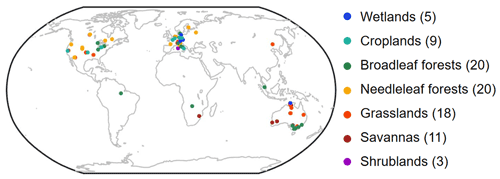Figure 1Location of the 86 flux sites used in this study. Numbers in the brackets indicate the number of sites for each biome type.

2 Materials and methods

## 2.1 Flux site observations

Observations of actual daily evaporation (or latent heat flux), sensible heat flux, and ground heat flux along with relevant meteorological variables, radiative fluxes and soil moisture were originally obtained from 212 flux sites collected in the FLUXNET2015 database (http://fluxnet.fluxdata.org/data/fluxnet2015-dataset/, last access: 2 November 2020). Only days with the data quality metric for LE and H higher than 0.9 (on a scale of 0–1) were used. The daily scale variables were obtained based on 15 min/30 min observations using the standard approach (Pastorello et al., 2020). The residual approach (i.e., assuming the observed H is correct and considering LE as the residual of the energy balance equation) was used to recalculate the fluxes based on a forced energy balance closure at each flux site (Ershadi et al., 2014). We also used the Bowen ratio approach (Twine et al., 2000) to force the flux-site energy balance closure and this resulted in similar model performance (Fig. S1 in the Supplement). The surface temperature for each site-day combination was calculated based on the observed longwave radiation following:

$\begin{array}{}\text{(2)}& {T}_{\mathrm{s}}=\sqrt[\mathrm{4}]{\frac{{R}_{\mathrm{lo}}-\left(\mathrm{1}-\mathit{\epsilon }\right){R}_{\mathrm{li}}}{\mathit{\epsilon }\mathit{\sigma }}},\end{array}$

where Rlo and Rli are, respectively, the outgoing and incoming longwave radiation, σ is the Stefan–Boltzmann constant ($\mathrm{5.67}×{\mathrm{10}}^{-\mathrm{8}}$ W m−2 K−4), and ε is the surface emissivity, which is acquired from the MODIS (Moderate Resolution Imaging Spectroradiometer) emissivity product (i.e., MOD11A1 Version 6; https://lpdaac.usgs.gov/products/mod11a1v006, last access: 2 January 2021). The MOD11A1 surface emissivity has a daily temporal resolution and a 1 km spatial resolution. To obtain the emissivity for each eddy covariance (EC) flux site, we center on the pixel where the site is located and take the mean value of the 81 neighboring pixels (9×9 pixels) as the emissivity value of the site. For conditions when the MOD11A1 emissivity are not available, we deleted these site-days.

To select a subset of observations at each flux site in which the actual evaporation is not limited by water availability, the energy balance criterion and the soil moisture criterion used by Maes et al. (2019) were adopted. Specifically, at each flux site, the evaporative fraction (EF; i.e., $\mathrm{EF}=LE/\left(LE+H\right)\right)$ was first calculated and the unstressed measurements consisted of all days with EF exceeding the 95th percentile EF threshold at each site. Following that, we removed days with soil moisture (averaged over all measured depths) lower than 50 % of the maximum soil moisture (taken to be soil moisture at the 98th percentile) at each site. In addition, any remaining site-days with daily EF values lower than 0.6 were also removed. Finally, we removed days having a negative H value (accounting for ∼5 % of the total daily data) to avoid dealing with strongly advective conditions when accurate measurements are not guaranteed (Paw U et al., 2000; Wilson et al., 2002). As a result, a total of 1128 non-water-stressed site-days from 86 sites passed the above criteria and were used in this study (Fig. 1 and Table S1 in the Supplement).

## 2.2 Maximum evaporation model

### 2.2.1 Overview of the maximum evaporation model

The maximum evaporation model calculates evaporation from a wet surface based essentially on the surface energy balance (Eq. 1), with Rn and β both explicitly represented as functions of Ts (Yang and Roderick, 2019):

$\begin{array}{}\text{(3)}& LE=\frac{\mathrm{1}}{\mathrm{1}+\mathit{\beta }\left({T}_{\mathrm{s}}\right)}\left[{R}_{\mathrm{n}}\left({T}_{\mathrm{s}}\right)-G\right].\end{array}$

In the above equation, the first term on the right-hand side (i.e., $\mathrm{1}/\left[\mathrm{1}+\mathit{\beta }\left({T}_{\mathrm{s}}\right)\right]$) is the evaporative fraction, which is the ratio of the latent heat flux over the total available energy. Over wet surfaces, since the Bowen ratio decreases with Ts (Aminzadeh et al., 2016; Andreas et al., 2013; Guo et al., 2015; Philip, 1987; Priestley and Taylor, 1972; Slatyer and McIlroy, 1961; Yang and Roderick, 2019), evaporative fraction increases with Ts. On the other hand, the second term on the right-hand side of Eq. (3) is the total available energy, which decreases with the increase of Ts as a higher Ts directly leads to a higher outgoing longwave radiation and hence a lower Rn (Yang and Roderick, 2019). As a result, the trade-off between a higher evaporative fraction and a lower Rn with the increase of Ts would naturally lead to a maximum LE along the Ts gradient according to Eq. (3). A previous study by Yang and Roderick (2019) demonstrated that this naturally emergent maximum LE corresponds well to the actual LE over global ocean surfaces and the Ts at which the maximum LE occurs also corresponds to the observed sea surface temperature. Here we will test whether this maximum evaporation approach is also valid over land under non-water-stressed conditions.

### 2.2.2 Parameterization of Rn and β as a function of Ts

To explicitly acknowledge the dependence of Rn on Ts, Rn(Ts) is expressed as

$\begin{array}{}\text{(4)}& {R}_{\mathrm{n}}\left({T}_{\mathrm{s}}\right)={R}_{\mathrm{sn}}+\mathit{\epsilon }\mathit{\sigma }{\left({T}_{\mathrm{s}}-\mathrm{\Delta }T\right)}^{\mathrm{4}}-\mathit{\epsilon }\mathit{\sigma }{T}_{\mathrm{s}}^{\mathrm{4}},\end{array}$

where Rsn is the net shortwave radiation (W m−2) and is taken to be unchanged with Ts. ΔT is the temperature difference between Ts and the effective radiating temperature of the atmosphere (Trad; assuming blackbody radiation, ${T}_{\mathrm{rad}}=\sqrt[\mathrm{4}]{{R}_{\mathrm{li}}/\mathit{\sigma }}\right)$, and is parameterized as a function of atmospheric transmissivity and geographic latitude (Yang and Roderick, 2019):

$\begin{array}{}\text{(5)}& \mathrm{\Delta }T={n}_{\mathrm{1}}\mathrm{exp}\left({n}_{\mathrm{2}}\mathit{\tau }\right)+{n}_{\mathrm{3}}\left|\mathrm{lat}\right|,\end{array}$

where τ is the atmospheric transmissivity for shortwave radiation (dimensionless) and is calculated as the ratio of incoming shortwave radiation at the Earth's surface to that at the top of the atmosphere. The parameter “lat” is the geographic latitude (in decimal degrees), which is considered here to account for a longer pathway of shortwave radiation going through the atmosphere in higher latitudes compared to lower latitudes. n1, n2, and n3 are the fitting coefficients. Using extensive data over the global ocean (n=202 794), Yang and Roderick (2019) determined the values of these coefficients to be n1=2.52, n2=2.38, and n3=0.035, respectively. Here, we directly adopt these same coefficient values over land for two reasons: (i) the key processes governing the interactions between incoming and outgoing longwave radiation are essentially the same for ocean and land (mainly greenhouse gases that affect the vertical temperature structure of the atmosphere, and water vapor and aerosols that affect the formation of clouds), and (ii) there were many more samples available for parameterizing Eq. (5) over the ocean than that over land. Validation against observations from all 1128 non-water-limited site-days demonstrates an overall good performance of Eq. (5) in estimating ΔT over land under saturated conditions (Fig. S2).

The Bowen ratio (β) is expressed as a function of Ts:

$\begin{array}{}\text{(6)}& \mathit{\beta }\left({T}_{\mathrm{s}}\right)=m\frac{\mathit{\gamma }\left({T}_{\mathrm{s}}\right)}{\mathrm{\Delta }\left({T}_{\mathrm{s}}\right)},\end{array}$

where m is a fitting coefficient. γ is the psychrometric constant (kPa K−1), and Δ is the slope of the saturation vapor pressure curve (kPa K−1), both of which are functions of Ts:

$\begin{array}{}\text{(7)}& & \mathit{\gamma }\left({T}_{\mathrm{s}}\right)=\frac{{C}_{\mathrm{P}}{P}_{\mathrm{a}}}{\mathrm{0.622}L\left({T}_{\mathrm{s}}\right)},\text{(8)}& & \mathrm{\Delta }\left({T}_{\mathrm{s}}\right)=\frac{\mathrm{4098}{e}_{\mathrm{s}}\left({T}_{\mathrm{s}}\right)}{{\left({T}_{\mathrm{s}}-\mathrm{35.8}\right)}^{\mathrm{2}}},\end{array}$

where CP is the specific heat of air at constant pressure (1.01 kJ kg−1 K−1), Pa is the air pressure (kPa), and es is the saturated vapor pressure (kPa). L is the latent heat of vaporization (kJ kg−1) and is calculated as a weak function of temperature:

$\begin{array}{}\text{(9)}& L\left({T}_{\mathrm{s}}\right)=\mathrm{2.51}×{\mathrm{10}}^{\mathrm{3}}-\mathrm{2.32}×\left({T}_{\mathrm{s}}-\mathrm{273.15}\right).\end{array}$

To apply the maximum evaporation model, an array of Ts (e.g., from 250 to 330 K at an interval of 0.1 K) is generated along with the observed Rsn and G; these are applied to Eqs. (4) and (6) and then Eq. (3) to estimate LE at each corresponding Ts. The maximum evaporation is then located in array as well as the surface temperature at which this maximum occurs (see Fig. 3 for an example).

3 Results

The maximum evaporation theory is tested at 86 flux sites globally, covering a wide range of bio-climates (Fig. 1 and Table S1). By pooling daily observations of H, LE, and Ts across all 1128 site-days, we first obtain a generic βTs relationship as $\mathit{\beta }=\mathrm{0.27}\mathit{\gamma }/\mathrm{\Delta }$. Similarly, we also obtained a βTs relationship for each separate biome type as shown in Fig. 2. By comparison, Yang and Roderick (2019) reported a βTs relationship over ocean of $\mathit{\beta }=\mathrm{0.24}\mathit{\gamma }/\mathrm{\Delta }$. This means that for the same Ts, β over land is generally larger than that over ocean. Interestingly, the ocean surface βTs relationship is identical to that in wetlands obtained here. These βTs relationships will be used in the following calculations of LE using the maximum evaporation approach.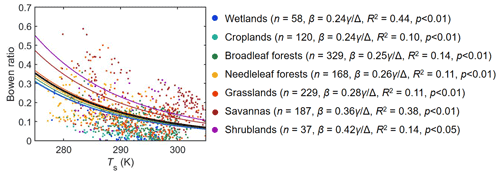Figure 2Relationship between Bowen ratio (β) and surface temperature (Ts) over saturated land surfaces. The thick black curve represents the fitted βTs relationship across all data points (i.e., n=1128, $\mathit{\beta }=\mathrm{0.27}\mathit{\gamma }/\mathrm{\Delta }$, R2=0.11, p<0.001), and the colored lines represent different biome types with the number of data points (n site-days) and fitted βTs relationship for each biome type shown in the legend.

To get an overview of how each of the energy fluxes varies with Ts, we first examine the maximum evaporation theory using the pooled data over all 1128 site-days (Fig. 3). Under this condition, the mean observed net shortwave radiation (Rsn) over all site-days is 176.6 W m−2 and G is 1.0 W m−2. Since Rsn is not directly dependent on Ts and G is negligibly small, the term Rsn minus G is held constant across the entire Ts range. With the increase of Ts, it is readily apparent that both outgoing and incoming longwave radiation (Rlo and Rli) steadily increase (see Sect. 2.2 for details about the coupling between Rlo and Rli), with Rlo increasing slightly faster than Rli, leading to a decreased net longwave radiation and thus a decreased Rn as Ts increases (Fig. 3). With this and the observed generic dependence of β on Ts ($\mathit{\beta }=\mathrm{0.27}\mathit{\gamma }/\mathrm{\Delta }$, Fig. 2), a maximum LE emerges along the Ts gradient that represents the interaction between decreasing Rn and increasing evaporative fraction as Ts increases. For the pooled dataset used here, the maximum LE (LEmax) is found to be 105.6 W m−2 and the corresponding Ts is 294.7 K, both of which are very close to the averages computed from all daily flux site observations (i.e., LEobs=102.4 W m−2 and Ts_obs=292.3 K) (Fig. 3).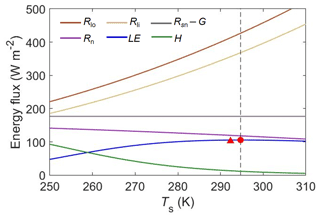Figure 3Variation of energy fluxes with Ts. Plot shows how the energy fluxes vary with Ts for a fixed value of RsnG at 176.6 W m−2 (Rsn is the net shortwave radiation; see Eq. 4 in Sect. 2.2). The red dot indicates the maximum evaporation and the red triangle shows the observed evaporation. The Ts at which the maximum evaporation occurs is shown by the dashed vertical line.

Having demonstrated the overall concept, we next perform the detailed calculations using data for all individual site-days (Figs. 4–6). Using the same generic β dependence on Ts ($\mathit{\beta }=\mathrm{0.27}\mathit{\gamma }/\mathrm{\Delta }$), the LEmax estimated from the maximum evaporation model agrees very well with flux site observations, yielding an R2 of 0.92, a root-mean-squared error (RMSE) of 14.6 W m−2, and a mean bias of 1.6 W m−2 (Fig. 4a). The performance of the maximum evaporation model improves slightly when the biome-specific model parameters are used (RMSE decreases to 14.1 W m−2 and mean bias decreases to 1.4 W m−2; Fig. 4b). This result demonstrates that LEmax corresponds to the observed evaporation under well-watered conditions across a broad range of bio-climates. In fact, when the previously identified ocean surface βTs relationship is adopted, the maximum evaporation approach performs only slightly worse than those based on the calibrated βTs relationship over saturated lands, yielding an R2 of 0.91, an RMSE of 14.8 W m−2, and a mean bias of 2.8 W m−2 (Fig. 4c).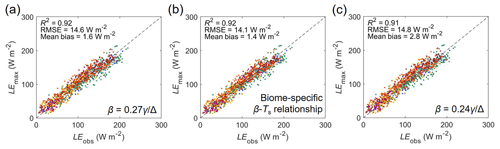Figure 4Comparison of the maximum evaporation and observed evaporation over saturated land surfaces using three different βTs relationships. (a) Generic land βTs relationship ($\mathit{\beta }=\mathrm{0.27}\mathit{\gamma }/\mathrm{\Delta }$, n=1128). (b) Biome-specific βTs relationships (per Fig. 2). (c) Ocean surface βTs relationship ($\mathit{\beta }=\mathrm{0.24}\mathit{\gamma }/\mathrm{\Delta }$, Yang and Roderick, 2019). The colors indicate different biome types (as provided in Fig. 1). The dashed black line indicates the 1:1 line.

We next test whether the maximum evaporation approach could recover Ts over the same saturated land surfaces. Similar to the test of LE, the three βTs relationships are respectively used. Results show that when the generic βTs relationship over land is used (i.e., $\mathit{\beta }=\mathrm{0.27}\mathit{\gamma }/\mathrm{\Delta }$), the Ts at which LEmax occurs corresponds reasonably well to the observed Ts, with an R2 of 0.62, an RMSE of 4.3 K, and a mean bias of 0.3 K (Fig. 5a), indicating that the maximum evaporation approach is also able to recover Ts under saturated conditions. Again, the model's performance in recovering Ts increases slightly when the biome-specific βTs relationships are used (Fig. 5b). When the ocean surface βTs relationship is used, the model performs similarly in estimating the variability of Ts to that of the generic land βTs relationship (Fig. 5c). However, the ocean surface βTs relationship (Fig. 5c) results in a higher Ts mean bias compared to the βTs relationships obtained over land (Fig. 5a).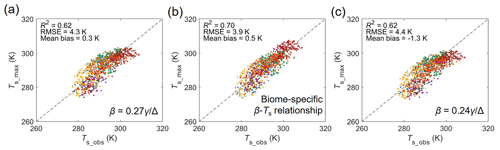Figure 5Comparison of the estimated and observed surface temperature over saturated land surfaces using three different βTs relationships. Comparison of estimated surface temperature (Ts_max) with flux site observations (Ts_obs). (a) Generic land βTs relationship ($\mathit{\beta }=\mathrm{0.27}\mathit{\gamma }/\mathrm{\Delta }$, n=1128). (b) Biome-specific βTs relationships (per Fig. 2). (c) Ocean surface βTs relationship ($\mathit{\beta }=\mathrm{0.24}\mathit{\gamma }/\mathrm{\Delta }$, Yang and Roderick, 2019). The colors indicate different biome types (as provided in Fig. 1). The dashed black line indicates the 1:1 line.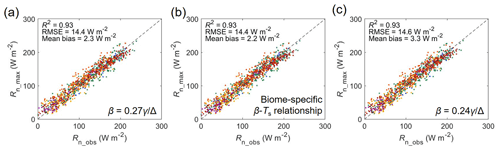Figure 6Comparison of the estimated and observed net radiation over saturated land surfaces using three different βTs relationships. Comparison of estimated net radiation (Rn_max) with flux site observations (Rn_obs). (a) Generic land βTs relationship ($\mathit{\beta }=\mathrm{0.27}\mathit{\gamma }/\mathrm{\Delta }$, n=1128). (b) Biome-specific βTs relationships (per Fig. 2). (c) Ocean surface βTs relationship ($\mathit{\beta }=\mathrm{0.24}\mathit{\gamma }/\mathrm{\Delta }$, Yang and Roderick, 2019). The colors indicate different biome types (as provided in Fig. 1). The dashed black line indicates the 1:1 line.

Different from most state-of-the-art evaporation models, the maximum evaporation approach does not rely on observed Rn (or independent Rn estimates) as model input but estimates Rn as a result of the RnTsE interaction. Here, we also test the estimated Rn calculated using the maximum evaporation approach as the discrepancy between LEmax and LEobs is mainly caused by the slight difference between Ts_max and Ts_obs that leads to different Rlo and Rli (and thus a different Rn) being used in the calculation of LEmax. It should be noted that since the observed shortwave radiation is used in the maximum evaporation model, validation of Rn is essentially the same as the validation of net longwave radiation. We find that the maximum evaporation model could satisfactorily reproduce the observed Rn when the generic land βTs relationship is used, as indicated by an R2 of 0.93, an RMSE of 14.4 W m−2, and a mean bias of 2.3 W m−2 (Fig. 6a). Using biome-specific βTs relationships or the ocean surface βTs relationship does not considerably increase or decrease the model's performance in estimating Rn (Fig. 6b and c).

4 Discussion

Taking Rn and/or Ts (or near-surface air temperature) to be independent forcings has long been identified as a scientific concern in the use of evaporation models (Milly, 1991; Monteith and Unsworth, 2013; Philip, 1987). Here, we test a maximum evaporation theory developed over the global ocean surface that addresses this concern by explicitly acknowledging the interdependence between radiation, surface temperature, and evaporation (Yang and Roderick, 2019). Our new results show that there exists a maximum evaporation along the Ts gradient that corresponds to the observed evaporation under saturated conditions over land (Figs. 3 and 4). In addition, the Ts at which LEmax occurs also corresponds reasonably well to the observed Ts (Figs. 3 and 5). These results mirror those found previously over the global ocean (Yang and Roderick, 2019). This is not a surprise since the basic principles are the same for a wet land surface and the ocean surface. These results suggest that saturated land surfaces behave essentially the same as ocean surfaces and imply that LEmax is a natural attribute of the land surface when water availability does not limit evaporation.

A key assumption involved in the maximum evaporation model is that β decreases with the increase of Ts under saturated conditions. Nevertheless, this key assumption that β decreases with the increase of Ts under saturated conditions has been extensively validated in previous studies based on theoretical relationships (Philip, 1987; Priestley and Taylor, 1972; Slatyer and McIlroy, 1961; Lhomme, 1997) and in situ observations (Andreas et al., 2013; Guo et al., 2015; Yang and Roderick, 2019; also see Fig. S3). Moreover, our results also found this held over saturated lands (Fig. 2). The original maximum evaporation study reported that $\mathit{\beta }=\mathrm{0.24}\mathit{\gamma }/\mathrm{\Delta }$ over global ocean surfaces (Yang and Roderick, 2019). Here, we find the generic land surface coefficient increases to 0.27 (i.e., $\mathit{\beta }=\mathrm{0.27}\mathit{\gamma }/\mathrm{\Delta }$, Fig. 2), which indicates a slightly higher β over wet vegetated land than that over the ocean surface for the same Ts. This is biophysically reasonable, as the stoma of plant leaves represents an additional resistance to vapor transfer between the land and the atmosphere (Swann et al., 2016; Yang et al., 2019), which lowers the ability of a generic vegetated surface to convert available energy into LE for a given Ts, compared to open water surfaces. In addition, different surface roughness can also lead to different βTs relationships between the land and the ocean. Compared with the ocean surface that shows a tight βTs relationship (Yang and Roderick, 2019), the βTs relationships over saturated vegetated land are relatively weak with considerable scatter (Fig. 2). This data scatter could be caused by several reasons. First, the observations by EC towers can be a source of uncertainty. This is threefold, including (i) the quality of the observations, (ii) the footprint within each EC tower (possibly) being heterogeneous (Lee et al., 2004; Paw et al., 2000), and (iii) whether or not the selected days are truly non-water-limited (however, see Fig. S4). Second, as is seen in Fig. 2, different biome types exhibit different βTs relationships. This can be caused by different surface resistance and roughness between biome types and even between sites. Nevertheless, these data-based limitations only have limited impacts on the model performance, as similar performance is obtained using both the generic βTs relationship (i.e., $\mathit{\beta }=\mathrm{0.27}\mathit{\gamma }/\mathrm{\Delta }$) and biome-specific βTs relationships (Fig. 4). Third, wind speed could be another factor that leads to the scatter. For the same surface roughness, a different wind speed will lead to a different aerodynamic resistance and therefore a different β. However, this effect is usually very small, as demonstrated by the long-standing similarity theory (the transfer of mass and heat share the same aerodynamic process in the lower atmospheric boundary layer; Monin and Obukhov, 1954). In fact, our findings that one can make a reasonable estimate of LE using a generic land or ocean βTs relationship instead of a site-specific relationship (Fig. 4) imply that Rn is the primary determinant of LE over saturated surfaces. As evaporation tends to operate at its maximum strength, sensible heat (and β) are usually very small over warm saturated land surfaces. As a result, once Rn can be accurately determined, any reasonable βTs relationship (Fig. 2) would result in a satisfactory LE estimate (Figs. 4 and S5). Our result highlighted in Fig. 3 shows that Rn (and hence LE) is only a weak function of Ts and this explains why one can obtain an accurate estimate of LE using a generic βTs relation. However, the same logic also leads to the conclusion that an accurate βTsrelationship will be necessary to estimate Ts, since Ts is very sensitive to changes in LE (Fig. 3). In this regard, using the land βTs relationships (preferably site-specific relations) is preferable to a generic ocean surface relation (Fig. 5). To further demonstrate the above points, we conduct an uncertainty test by varying the coefficient m in the βTs relationship in Fig. S6. We find that when m ranges from 0.18 to 0.36 (all other forcings as per Fig. 3), the change in estimated LEmax is only 9 W m−2 (101.7–110.7 W m−2), whereas the change in estimated Ts_max is as high as 11.6 K (287.9–299.5 K).

Besides the data scattering that leads to an uncertainty in the βTs relationships, there are also uncertainties associated with (i) parameterization of the longwave coupling and (ii) selection of non-water-stressed observations in the current study. In the maximum evaporation approach, the coupling between outgoing and incoming longwave radiation is calculated using the temperature difference between the surface and an effective radiating height in the atmosphere (ΔT) and is parameterized as a function of shortwave atmospheric transmissivity and geographic latitude. However, the shortwave atmospheric transmissivity is primarily affected by aerosols, while the longwave transmissivity is mainly affected by the concentration of greenhouse gases. Nevertheless, here we only deal with wet conditions, under which the vapor concentration of the atmosphere is also relatively high and more aerosols would favor the development of more clouds that simultaneously affect both shortwave and longwave radiation. We suspect that this underlies the excellent performance of Eq. (5) in estimating ΔTat the flux sites (Fig. S2). To further evaluate that conclusion, we additionally evaluate the estimated longwave radiation against four global products (i.e., ERA5, Hersbach et al., 2020; CERES, Kato et al., 2018; the Princeton global forcing data, Sheffield et al., 2006; the GLDAS global forcing data, Rodell et al., 2004) and compare our longwave estimates with other two semi-empirical models (i.e., Brutsaert, 1975; Shakespeare and Roderick, 2021). The results show our ΔT-based approach to be the best performer across a wide range of conditions when the surface is wet (Fig. S7). In addition, we further note that our maximum evaporation model is only tested at the daily timescale (Figs. 4–6) and longer (Fig. 3). In particular, for timescales shorter than that (e.g., hourly), the diurnal cycle of E can be very different for ocean and land surfaces (Kleidon and Renner, 2017). In addition, the parameterization of the coupling between incoming and outgoing longwave radiation via Eq. (5) requires a timescale that is long enough to allow the surface heat fluxes to be fully redistributed through the atmospheric column (Yang and Roderick, 2019). At sub-daily scales, Eq. (5) is likely invalid because Rlo usually exhibits a larger diurnal range than Rli during a typical cloudless day (Monteith and Unsworth, 2013). For even longer periods, especially for assessing the impacts of climate change, the relationship between shortwave and longwave radiation used herein may also be invalid, as we expect this relationship to evolve with anthropogenic climate change.

As for the selection of non-water-stressed evaporation observations from global EC towers, we rely largely on the same selection criteria used in a previous study (Maes et al., 2019). However, these selection criteria are somewhat subjective and represent a compromise between better data quality and more data samples. As a result, the selected site-days are not necessarily non-water-limited. Nevertheless, varying the selection criteria (changing the thresholds) of non-water-stressed evaporation only resulted in minor changes in the overall model performance (Fig. S4), which suggests that the uncertainties in the selection of non-water-stressed evaporation observations would not materially change our conclusion.

The ability of the maximum evaporation model to recover LE and Ts over vegetated lands under saturated conditions has an important implication for the estimation of potential evaporation, which is a central concept in hydrology and agriculture (and especially in irrigation). The underlying idea of EP is straightforward – it is the evaporation that would occur with an unlimited supply of water. However, the formal physical definition of EP has been widely debated in the literature (Brutsaert, 2005; Donohue et al., 2010; Granger, 1989; Nash, 1989) and the calculation of EP using conventional evaporation models is problematic (Aminzadeh et al., 2016; Roderick et al., 2015). The key scientific issue is that the meteorological forcing variables observed over actual surfaces are generally not equivalent to the meteorological variables that would be measured over a hypothetical surface with an unlimited water supply. Compared with existing evaporation models, the maximum evaporation model presented here requires fewer meteorological variables than existing approaches (but performs similarly with existing approaches under wet conditions; see Fig. S8 for details). This new approach only requires the incoming and reflected solar radiation, a relationship that describes a decreasing dependence of β on Ts, and a relation for the coupling of the incoming and outgoing longwave radiation. With these modest requirements, LEmax naturally follows from the physical interdependence between radiation, surface temperature, and evaporation. These features suggest that the maximum evaporation model can be used to make a strictly independent estimate of EP. In fact, the maximum evaporation formulation directly maps to one particular definition of EP that was proposed by Brutsaert (2015) as “the maximum evaporation that would occur over real surfaces with the actual solar forcing and a prescribed Bowen ratio”.

5 Conclusions

In this study, we test a maximum evaporation theory that explicitly acknowledges the interdependence between radiation, surface temperature, and evaporation over saturated land surfaces. Validated against flux site observations, we show that the maximum evaporation approach could recover the observed evaporation across a broad range of bio-climates. In comparison, although the model is also able to reasonably recover the observed Ts, the model's performance in recovering Ts is not as good as that for LE. Nevertheless, this does not materially lead to larger errors in LE estimates, as we additionally demonstrate that LE is not sensitive to Ts changes. The overall good performance of the maximum evaporation approach over saturated surfaces implies the method's great potential for use in estimating potential evaporation. To calculate EP in practice using the maximum evaporation approach, a detailed site-specific (or biome-specific) βTs relationship (e.g., Fig. 2) would be favorable; otherwise, a generic default βTs relationship ($\mathit{\beta }=\mathrm{0.27}\mathit{\gamma }/\mathrm{\Delta }$ or even $\mathit{\beta }=\mathrm{0.24}\mathit{\gamma }/\mathrm{\Delta }$) can also lead to a reasonable EP estimate that remains consistent with the EP definition by Brutsaert (2015). Table S2 gives a working example of applying the maximum evaporation model for EP estimation.

Data availability

All data for this paper are properly cited and referred to in the reference list. The FLUXNET2015 dataset is acquired from http://fluxnet.fluxdata.org/data/fluxnet2015-dataset/ (FLUXNET, 2016).

Supplement

Author contributions

YY and MLR conceived the idea and designed the research. ZT performed the calculation. YY drafted the manuscript. All authors contributed to the results, discussion, and manuscript writing.

Competing interests

The contact author has declared that neither they nor their co-authors have any competing interests.

Disclaimer

Publisher's note: Copernicus Publications remains neutral with regard to jurisdictional claims in published maps and institutional affiliations.

Acknowledgements

Michael L. Roderick acknowledges the support of the Australian Research Council (DP190100791). The FLUXNET community is greatly appreciated for making the eddy covariance data publicly available.

Financial support

This study is financially supported by the National Natural Science Foundation of China (grant nos. 42041004, 42071029, 41890821) and the Ministry of Science and Technology of China (grant no. 2019YFC1510604).

Review statement

This paper was edited by Adriaan J. (Ryan) Teuling and reviewed by Miriam Coenders-Gerrits and two anonymous referees.

References

Allen, R. G., Pereira, L. S., Raes, D., and Smith, M.: Crop Evapotranspiration – Guidelines for computing crop water requirements, FAO Irrigation and Drainage Paper No. 56, FAO – Food and Agriculture Organization of the United Nations, Rome, Italy, https://www.fao.org/3/X0490E/x0490e00.htm#Contents (last access: 4 April 2022), 1998.

Aminzadeh, M., Roderick, M. L., and Or, D.: A generalized complementary relationship between actual and potential evaporation defined by a reference surface temperature, Water. Resour. Res., 52, 385–406, https://doi.org/10.1002/2015WR017969, 2016.

Andreas, E. L., Jordan, R. E., Mahrt, L., and Vickers, D.: Estimating the Bowen ratio over the open and ice-covered ocean, J. Geophys. Res.-Oceans, 118, 4334–4345, https://doi.org/10.1002/jgrc.20295, 2013.

Bowen, I. S.: The ratio of heat losses by conduction and by evaporation from any water surface, Phys. Rev., 27, 779, https://doi.org/10.1103/PhysRev.27.779, 1926.

Brutsaert, W.: On a derivable formula for long-wave radiation from clear skies, Water. Resour. Res., 11, 742–744, https://doi.org/10.1029/WR011i005p00742, 1975.

Brutsaert, W.: Hydrology: an introduction, Cambridge University Press, Cambridge, UK, ISBN 9780521824798, 2005.

Brutsaert, W.: A generalized complementary principle with physical constraints for land-surface evaporation, Water. Resour. Res., 51, 8087–8093, https://doi.org/10.1002/2015WR017720, 2015.

Donohue, R. J., McVicar, T. R., and Roderick, M. L.: Assessing the ability of potential evaporation formulations to capture the dynamics in evaporative demand within a changing climate, J. Hydrol., 386, 186–197, https://doi.org/10.1016/j.jhydrol.2010.03.020, 2010.

Ershadi, A., McCabe, M. F., Evans, J. P., Chaney, N. W., and Wood, E. F.: Multi-site evaluation of terrestrial evaporation models using FLUXNET data, Agr. Forest Meteorol., 187, 46–61, https://doi.org/10.1016/j.agrformet.2013.11.008, 2014.

FLUXNET: FLUXNET2015 Dataset, http://fluxnet.fluxdata.org/data/fluxnet2015-dataset/ (last access: 12 November 2020), 2016.

Granger, R. J.: An examination of the concept of potential evaporation, J. Hydrol., 111, 9–19, https://doi.org/10.1016/0022-1694(89)90248-5, 1989.

Guo, X., Liu, H., and Yang, K.: On the application of the Priestley–Taylor relation on sub-daily time scales, Bound.-Lay. Meteorol., 156, 489–499, https://doi.org/10.1007/s10546-015-0031-y, 2015.

Hersbach, H., Bell, B., Berrisford, P., Hirahara, S., Horányi, A., Muñoz Sabater, J., Nicolas, J., Peubey, C., Radu, R., Schepers, D., Simmons, A., Soci, C., Abdalla, S., Abellan, X., Balsamo, G., Bechtold, P., Biavati, G., Bidlot, J., Bonavita, M., Chiara, G. D., Dahlgren, P., Dee, D., Diamantakis, M., Dragani, R., Flemming, J., Forbes, R., Fuentes, M., Geer, A., Haimberger, L., Healy, S., Hogan, R. J., Hólm, E., Janisková, M., Keeley, S., Laloyaux, P., Lopez, P., Lupu, C., Radnoti, G., Rosnay, P., Rozum, I., Vamborg, F., Villaume, S., and Thépaut, J.: The ERA5 global reanalysis, Q. J. Roy. Meteorol. Soc., 146, 1999–2049, https://doi.org/10.1002/qj.3803, 2020.

Jasechko, S., Sharp, Z. D., Gibson, J. J., Birks, S. J., Yi, Y., and Fawcett, P. J.: Terrestrial water fluxes dominated by transpiration, Nature, 496, 347–350, https://doi.org/10.1038/nature11983, 2013.

Kato, S., Rose, F. G., Rutan, D. A., Thorsen, T. J., Loeb, N. G., Doelling, D., Huang, X., Smith, W. L., Su, W., and Ham, S.: Surface irradiances of edition 4.0 Clouds and the Earth' s Radiant Energy System (CERES) Energy Balanced and Filled (EBAF) data product, J. Climate, 31, 4501–4527, https://doi.org/10.1175/JCLI-D-17-0523.1, 2018.

Kleidon, A. and Renner, M.: An Explanation for the Different Climate Sensitivities of Land and Ocean Surfaces Based on the Diurnal Cycle, Earth Syst. Dynam., 8, 849–864, https://doi.org/10.5194/esd-8-849-2017, 2017.

Lee, X., Massman, W., and Law, B.: Handbook of micrometeorology: a guide for surface flux measurement and analysis, Vol. 29, Springer Science & Business Media, ISBN 1402022646, 2004.

Lhomme, J. P.: A theoretical basis for the Priestley-Taylor coefficient, Bound.-Lay. Meteorol., 82, 179–191, https://doi.org/10.1023/A:1000281114105, 1997.

Lian, X., Piao, S., Huntingford, C., Li, Y., Zeng, Z., Wang, X., Ciais, P., McVicar, T., Peng, S., Ottlé, C., Yang, H., Yang, Y., Zhang, Y., and Wang, T.: Partitioning global land evapotranspiration using CMIP5 models constrained by observations, Nat. Clim. Change, 8, 640–646, 2018.

Maes, W. H., Gentine, P., Verhoest, N. E., and Miralles, D. G.: Potential evaporation at eddy-covariance sites across the globe, Hydrol. Earth. Syst. Sci., 23, 925–948, https://doi.org/10.5194/hess-23-925-2019, 2019.

Milly, P. C. D.: A refinement of the combination equations for evaporation, Surv. Geophys., 12, 145–154, https://doi.org/10.1007/BF01903416, 1991.

Milly, P. C. D. and Dunne, K. A.: Potential evapotranspiration and continental drying, Nat. Clim. Change, 6, 946–949, https://doi.org/10.1038/nclimate3046, 2016.

Monin, A. S. and Obukhov, A. M.: Basic laws of turbulent mixing in the surface layer of the atmosphere, Contrib. Geophys. Inst. Acad. Sci. USSR, 151, e187, 1954.

Monteith, J. L.: Evaporation and environment, Symp. Soc. Exp. Biol., 19, 205–234, 1965.

Monteith, J. L. and Unsworth, M.: Principles of environmental physics: plants, animals, and the atmosphere, Academic Press, San Diego, USA, ISBN 0123869102, 2013.

Nash, J. E.: Potential evaporation and “the complementary relationship”, J. Hydrol., 111, 1–7, https://doi.org/10.1016/0022-1694(89)90247-3, 1989.

Pastorello, G., Trotta, C., Canfora, E., Chu, H., Christianson, G., Cheah, Y., Poindexter, C., Chen, J., Elbashandy, A., Humphrey, M., Isaac, P., Polidori, D., Reichstein, M., Ribeca, A., Ingen, C., Vuichard, N., Zhang, L., Amiro, B., Ammann, C., Arain, M. A., Ardö, J., Arkebauer, T., Arndt, S. K., Arriga, N., Aubinet, M., Aurela, M., Baldocchi, D., Barr, A., Beamesderfer, E., Marchesini, L. B., Bergeron, O., Beringer, J., Bernhofer, C., Berveiller, D., and Billesbach, D.: The FLUXNET2015 dataset and the ONEFlux processing pipeline for eddy covariance data, Sci. Data, 7, 1–27, https://doi.org/10.1038/s41597-020-0534-3, 2020.

Paw U, K. T., Baldocchi, D. D., Meyers, T. P., and Wilson, K. B.: Correction of eddy-covariance measurements incorporating both advective effects and density fluxes, Bound.-Lay. Meteorol., 97, 487–511, https://doi.org/10.1023/A:1002786702909, 2000.

Penman, H. L.: Natural evaporation from open water, bare soil and grass, P. Roy. Soc. Lond. A, 193, 120–145, https://doi.org/10.1098/rspa.1948.0037, 1948.

Philip, J. R.: A physical bound on the Bowen ratio, J. Clim. Appl. Meteorol., 26, 1043–1045, https://doi.org/10.1175/1520-0450(1987)026<1043:APBOTB>2.0.CO;2, 1987.

Priestley, C. H. B. and Taylor, R. J.: On the assessment of surface heat flux and evaporation using large-scale parameters, Mon. Weather. Rev., 100, 81–92, https://doi.org/10.1175/1520-0493(1972)100<0081:OTAOSH>2.3.CO;2, 1972.

Rodell, M., Houser, P. R., Jambor, U., Gottschalck, J., Mitchell, K., Meng, C. J., Arsenault, K., Cosgrove, B., Radakovich, J., Bosilovich, M., Entin, J. K., Walker, J. P., Lohmann, D., and Toll, D.: The Global Land Data Assimilation System, B. Am. Meteorol. Soc., 85, 381–394, https://doi.org/10.1175/BAMS-85-3-381, 2004.

Roderick, M. L., Greve, P., and Farquhar, G. D.: On the assessment of aridity with changes in atmospheric CO2, Water. Resour. Res., 51, 5450–5463, https://doi.org/10.1002/2015WR017031, 2015.

Scheff, J. and Frierson, D. M. W.: Scaling potential evapotranspiration with greenhouse warming, J. Climate, 27, 1539–1558, https://doi.org/10.1175/JCLI-D-13-00233.1, 2014.

Schellekens, J., Dutra, E., Martinez-de la Torre, A., Balsamo, G., van Dijk, A., Weiland, F. S., Minvielle, M., Calvet, J. C., Decharme, B., Eisner, S., Fink, G., Florke, M., Pessenteiner, S., van Beek, R., Polcher, J., Beck, H., Orth, R., Calton, B., Burke, S., Dorigo, W., and Weedon, G. P.: A global water resources ensemble of hydrological models: the eartH2Observe Tier-1 dataset, Earth. Syst. Sci. Data, 9, 389–413, https://doi.org/10.5194/essd-9-389-2017, 2017.

Shakespeare, C. J. and Roderick, M. L.: The clear-sky downwelling longwave radiation at the surface in current and future climates, Q. J. Roy. Meteorol. Soc., 147, 4251–4268, https://doi.org/10.1002/qj.4176, 2021.

Sheffield, J., Goteti, G., and Wood, E. F.: Development of a 50-year high-resolution global dataset of meteorological forcings for land surface modeling, J. Climate, 19, 3088–3111, https://doi.org/10.1175/JCLI3790.1, 2006.

Sheffield, J., Wood, E. F., and Roderick, M. L.: Little change in global drought over the past 60 years, Nature, 491, 435–438, https://doi.org/10.1038/nature11575, 2012.

Shuttleworth, W. J.: Evaporation, in Handbook of Hydrology, chap. 4, edited by: Maidment, D. R., McGraw-Hill Education, New York, USA, ISBN 0070397325, 1993.

Slatyer, R. O. and McIlroy, I. C.: Practical microclimatology, Commonwealth Scientific and Industrial Research Organisation, Canberra, Australia, https://doi.org/10.1016/0002-1571(65)90036-1, 1961.

Swann, A. L., Hoffman, F. M., Koven, C. D., and Randerson, J. T.: Plant responses to increasing CO2 reduce estimates of climate impacts on drought severity, P. Natl. Acad. Sci. USA, 113, 10019–10024, https://doi.org/10.1073/pnas.1604581113, 2016.

Thornthwaite, C. W.: An approach toward a rational classification of climate, Geogr. Rev., 38, 55–94, https://doi.org/10.2307/210739, 1948.

Twine, T. E., Kustas, W. P., Norman, J. M., Cook, D. R., Houser, P. R., Meyers, T. P., Prueger, J. H., Srarks, P. J., and Wesely, M. L.: Correcting eddy-covariance flux underestimates over a grassland, Agr. Forest Meteorol., 103, 279–300, https://doi.org/10.1016/S0168-1923(00)00123-4, 2000.

Vicente-Serrano, S. M., Gouveia, C., Camarero, J. J., Beguería, S., Trigo, R., López-Moreno, J. I., Azorín-Molina, C., Pasho, E., Lorenzo-Lacruz, J., Revuelto, J., Morán-Tejeda, E., and Sanchez-Lorenzo, A.: Response of vegetation to drought time-scales across global land biomes, P. Natl. Acad. Sci. USA, 110, 52–57, https://doi.org/10.1073/pnas.1207068110, 2013.

Wang, K. and Dickinson, R. E.: A review of global terrestrial evapotranspiration: Observation, modeling, climatology, and climatic variability, Rev. Geophys., 50, RG2005, https://doi.org/10.1029/2011RG000373, 2012.

Wilson, K., Goldstein, A., Falge, E., Aubinet, M., Baldocchi, D., Berbigier, P., Bernhofer, C., Ceulemans, R., Dolman, H., Field, C., Grelle, A., Ibrom, A., Law, B. E., Kowalski, A., Meyers, T., Moncrieff, J., Monson, R., Oechel, W., Tenhunen, J., Valentini, R., and Verma, S.: Energy balance closure at FLUXNET sites, Agr. Forest Meteorol., 113, 223–243, https://doi.org/10.1016/S0168-1923(02)00109-0, 2002.

Yang, Y. and Roderick, M. L.: Radiation, surface temperature and evaporation over wet surfaces, Q. J. Roy. Meteorol. Soc., 145, 1118–1129, https://doi.org/10.1002/qj.3481, 2019.

Yang, Y., Roderick, M. L., Zhang, S., McVicar, T. R., and Donohue, R. J.: Hydrologic implications of vegetation response to elevated CO2 in climate projections, Nat. Clim. Change, 9, 44–48, https://doi.org/10.1038/s41558-018-0361-0, 2019.